## RS Aggarwal Class 9 Solutions Chapter 10 Area Ex 10A

These Solutions are part of RS Aggarwal Solutions Class 9. Here we have given RS Aggarwal Class 9 Solutions Chapter 10 Area Ex 10A.

Question 1.
Solution:
Given : In the figure, ABCD is a quadrilateral and
AB = CD = 5cm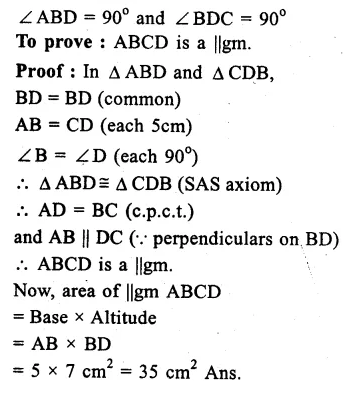Question 2.
Solution:
In ||gm ABCD,
AB = 10cm, altitude DL = 6cm
and BM is altitude on AD, and BM = 8 cm.Question 3.
Solution:
Diagonals of rhombus are 16cm and 24 cm.
Area = $$\frac { 1 }{ 2 }$$ x product of diagonals
= $$\frac { 1 }{ 2 }$$ x 1st diagonal x 2nd diagonal
= $$\frac { 1 }{ 2 }$$ x 16 x 24
= 192 cm² Ans.

Question 4.
Solution:
Parallel sides of a trapezium are 9cm and 6cm and distance between them is 8cm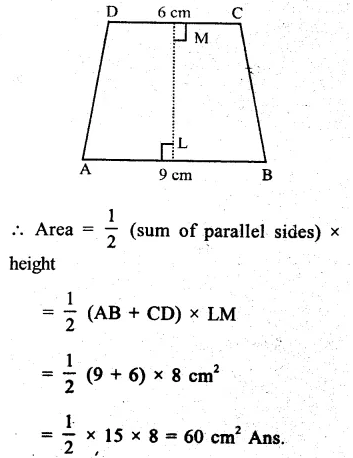Question 5.
Solution:
from the figure
(i) In ∆ BCD, ∠ DBC = 90°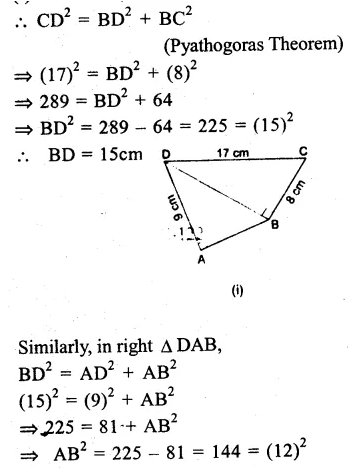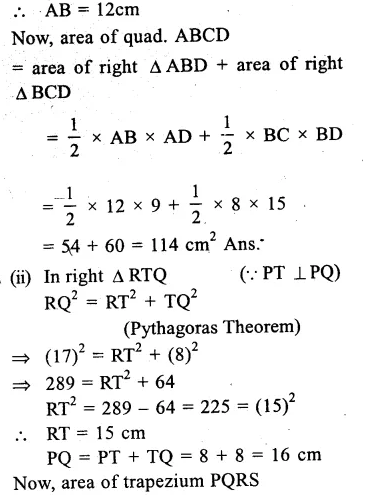Question 6.
Solution:
In the fig, ABCD is a trapezium. AB || DC
AB = 7cm, AD = BC = 5cm.
Distance between AB and DC = 4 cm.
i.e. ⊥AL = ⊥BM = 4cm.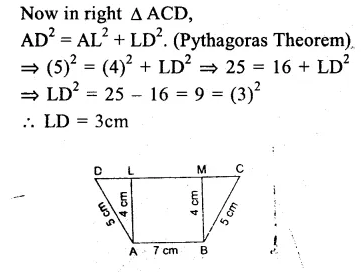Question 7.
Solution:
Given : In quad. ABCD. AL⊥BD and CM⊥BD.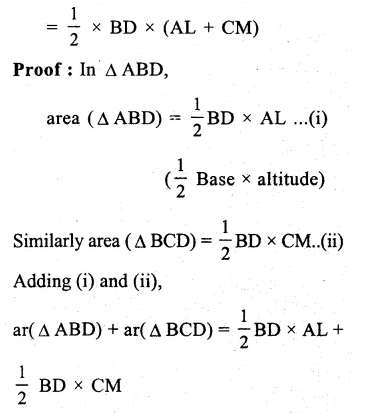Question 8.
Solution:
In quad. ABCD, BD is its diagonal and AL⊥BD, CM⊥BD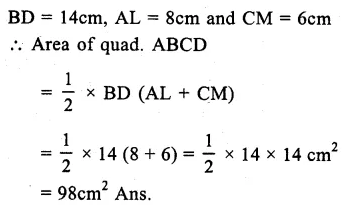Question 9.
Solution:
Given : ABCD is a trapezium in which AB || DC and its diagonals AC and BD intersect each other at O.
To prove : ar(∆ AOD) = ar(∆ BOC)Question 10.
Solution:
Given : In the figure,
DE || BC.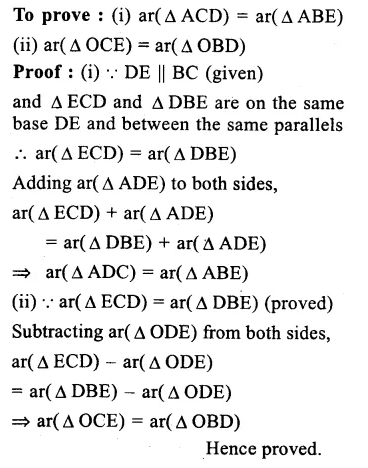Question 11.
Solution:
Given : In ∆ ABC, D and E are the points on AB and AC such that
ar( ∆ BCE) = ar( ∆ BCD)
To prove : DE || BC.
Proof : (∆ BCE) = ar(∆ BCD)
But these are on the same base BC.
Their altitudes are equal.
Hence DE || BC
Hence proved.

Question 12.
Solution:
Given : In ||gm ABCD, O is any. point inside the ||gm. OA, OB, OC and OD are joined.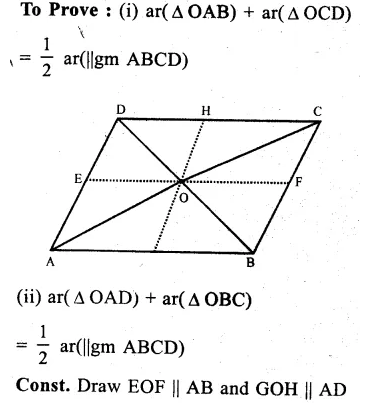Question 13.
Solution:
A line through D, parallel to AC, meets ‘BC produced in P. AP in joined which intersects CD at E.
To prove : ar( ∆ ABP) = ar(quad. ABCD).
Const. Join AC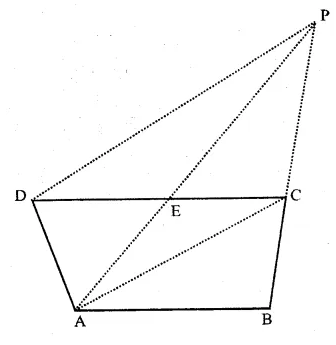Question 14.
Solution:
Given : ∆ ABC and ∆ DBC are on the same base BC with points A and D on , opposite sides of BC and
ar( ∆ ABC) = ar( ∆ DBC).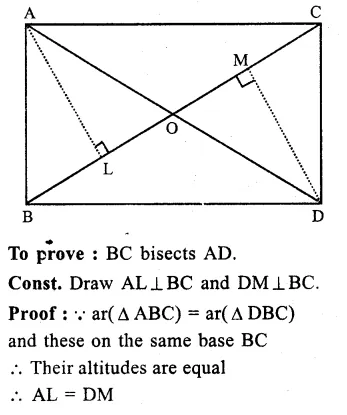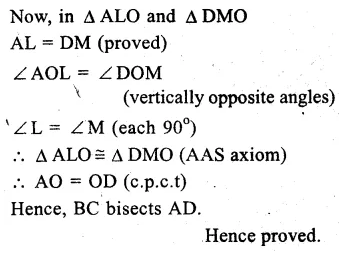Question 15.
Solution:
Given : In ∆ ABC, AD is the median and P is a point on AD
BP and CP are joined
To prove : (i) ar(∆BDP) = ar(∆CDP)
(ii) ar( ∆ ABP) = ar( ∆ ACP)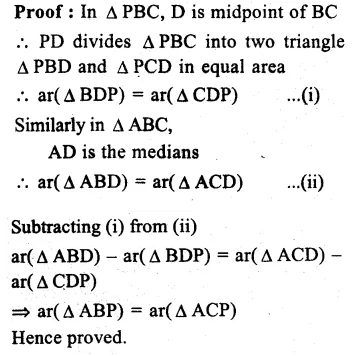Question 16.
Solution:
Given : In quad. ABCD, diagonals AC and BD intersect each other at O and BO = OD
To prove : ar(∆ ABC) = ar(∆ ADC)
Proof : In ∆ ABD,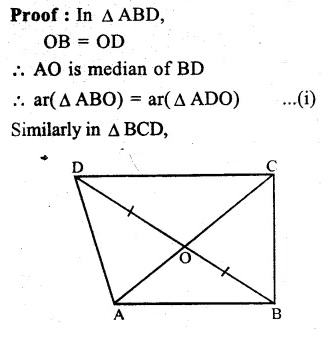Question 17.
Solution:
In ∆ ABC,D is mid point of BC
and E is midpoint of AD and BE is joined.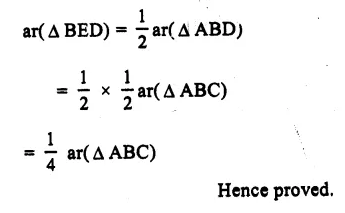Question 18.
Solution:
Given : In ∆ ABC. D is a point on AB and AD is joined. E is mid point of AD EB and EC are joined.
To prove : ar( ∆ BEC) = $$\frac { 1 }{ 2 }$$ ar( ∆ ABC)
Proof : In ∆ ABD,
BE is its median
ar(∆ EBD) = ar(∆ ABE)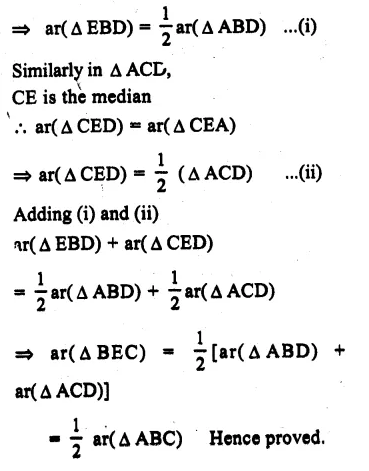Question 19.
Solution:
Given : In ∆ ABC, D is midpoint of BC and E is die midpoint of BO is the midpoint of AE.
To prove that ar( ∆ BOE) = $$\frac { 1 }{ 8 }$$ ar(∆ ABC).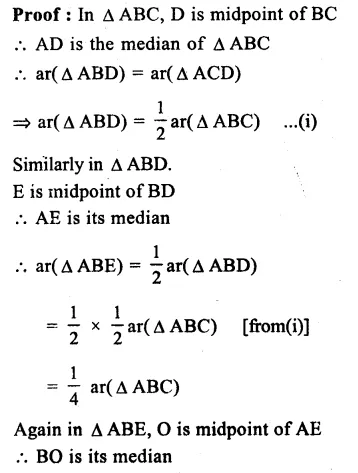Question 20.
Solution:
Given : In ||gm ABCD, O is any point on diagonal AC.
To prove : ar( ∆ AOB) = ar( ∆ AOD)
Const. Join BD which intersects AC at P
Proof : In ∆ OBD,
P is midpoint of BD
(Diagonals of ||gm bisect each other)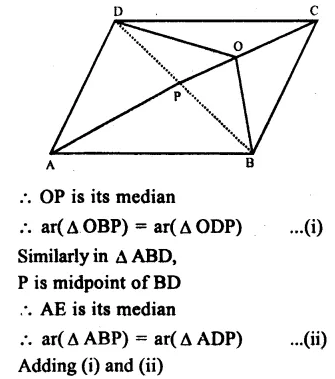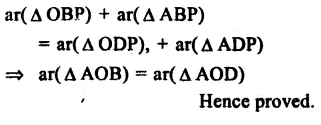Question 21.
Solution:
Given : ABCD is a ||gm.
P, Q, R and S are the midpoints of sides AB, BC, CD, DA respectively.
PQ, QR, RS and SP are joined.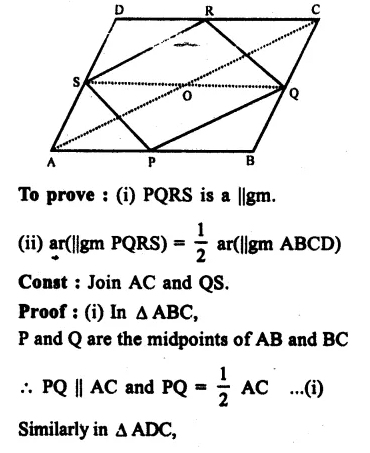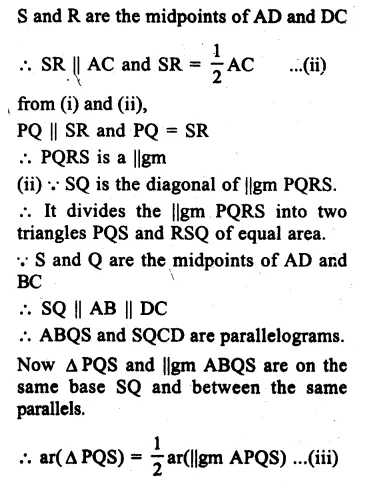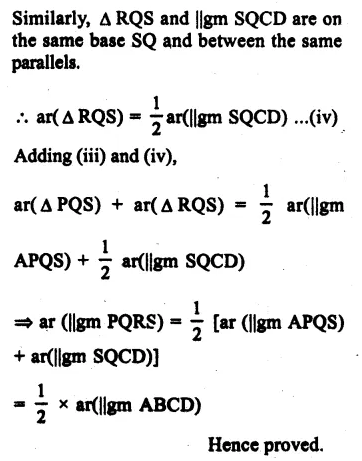Question 22.
Solution:
Given : In pentagon ABCDE,
EG || DA meets BA produced and
CF || DB, meets AB produced.
To prove : ar(pentagon ABCDE) = ar(∆ DGF)Question 23.
Solution:
Given ; A ∆ ABC in which AD is the median.
To prove ; ar( ∆ ABD) = ar( ∆ ACD)
Const : Draw AE⊥BC.
Proof : Area of ∆ ABD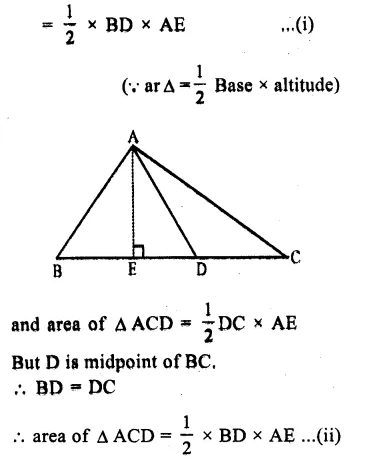Question 24.
Solution:
Given : A ||gm ABCD in which AC is its diagonal which divides ||gm ABCD in two ∆ ABC and ∆ ADC.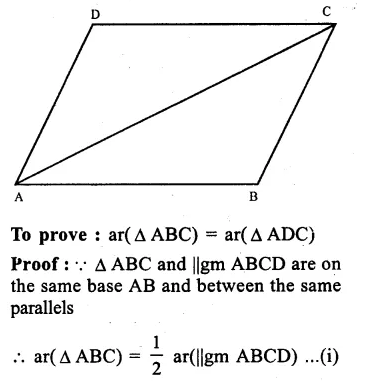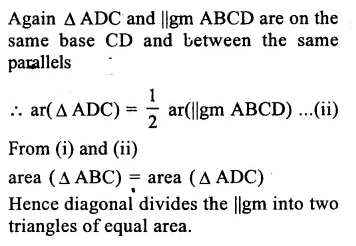Question 25.
Solution:
Given : In ∆ ABC,
D is a point on BC such that
BD = $$\frac { 1 }{ 2 }$$ DC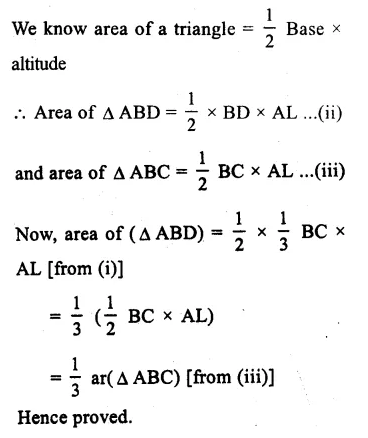Question 26.
Solution:
Given : In ∆ ABC, D is a point on BC such that
BD : DC = m : n